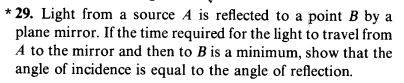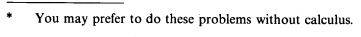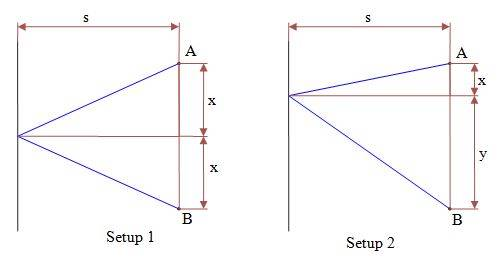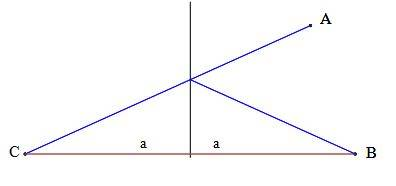# Min Max problem: the shortest distance for a light ray

## Homework Statement## Homework Equations

Minimum/Maximum occurs when the first derivative=0
GM≤AM: ##~\sqrt{xy}\leq\frac{x+y}{2}##

## The Attempt at a Solution[/B]
If the sum of squares of the distances (setup 2) in an arbitrary point is bigger than the sum of the squares of the shortest distances (setup 1, the mid point between A and B) then the distance from an arbitrary point is also bigger. i want to prove without calculus that:
$$2s^2+x^2+y^2\geq 2s^2+2\left[ \frac{x+y}{2} \right]~\rightarrow~\frac{3}{2}(x^2+y^2)\geq xy$$
I want to use GM≤AM so i take the square root of what i need to prove, and i need to prove:
$$\sqrt{1.5}\sqrt{x^2+y^2}\geq\sqrt{xy}$$
I know ##~\sqrt{xy}\leq\frac{x+y}{2}~## so i have to prove:
$$\sqrt{xy}\leq\frac{x+y}{2}\leq\sqrt{1.5}\sqrt{x^2+y^2}$$
And it remains that i have to prove:
$$\frac{x+y}{2}\leq\sqrt{1.5}\sqrt{x^2+y^2}$$
I know ##~\sqrt{(x+y)^2}=\sqrt{x^2+y^2+2xy}\leq\sqrt{x^2+y^2}~## but it doesn't help since i need to insert an inequality
on the left of ##~\sqrt{1.5}\sqrt{x^2+y^2}~## and not on the right side.

#### Attachments

You can use AM-QM

AM-QM, in my case, is exactly GM≤AM: ##~~\sqrt{xy}\leq\frac{x+y}{2}##
It doesn't help in proving ##~\frac{x+y}{2}\leq\sqrt{1.5}\sqrt{x^2+y^2}##

StoneTemplePython
Gold Member
Karol if you want to prove

##\frac{3}{2}(x^2+y^2)\geq xy##

first recognize that ##\frac{3}{2}(x^2+y^2) \geq \frac{1}{2}(x^2+y^2)##

now ##xy = \big(x^2 y ^2\big)^{\frac{1}{2}}= \sqrt{\big(x^2 y ^2\big)}##... right? What can you do with that?

LCKurtz
Homework Helper
Gold Member
I know ##\sqrt{x^2+y^2+2xy}\leq\sqrt{x^2+y^2}~##
You do? Try ##x=y=1##

$$\frac{3}{2}(x^2+y^2)\geq \frac{x^2+y^2}{2}\geq \sqrt{x^2y^2}=xy$$
But i am not sure i can use the same x for the two setups, 1 and 2.I want the shortest distance between A and B. AC is the shortest and equals the shortest AB

Last edited: